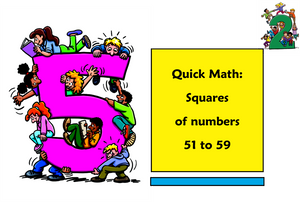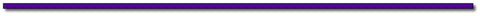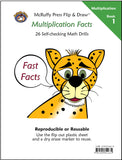# Quick Math: Squaring Numbers 51 to 59In our previous post, we found a quick shortcut to square two-digit numbers with 5 in the ones place. There is an even simpler shortcut to square two-digit numbers with the digit 5 in the tens place.

For younger students, you can simply teach the trick. For older students explore the Why It Works  section.

The Trick:

To get the thousands and hundreds place value add the digit in the ones place (units) to 25.

To get the tens and ones place, square the number in the ones place. The tens place will be zero for the numbers 51, 52, and 53.

For example, 542 Add the ones digit to 25 to get the thousands and hundreds places. 25 + 4 = 29, which represents 2900.

Square the digit in the ones place to get the digits for the tens and units. 4 x 4 = 16

542 = 2900 + 16 = 2916

Print the Teaching Sheets for the trick. On the first sheet ask students to put in a random digit in the box to complete the two-digit number to square. Follow the steps. Remind students that if the product of squaring the ones digit is a single digit answer, the tens place is zero.

The second page is a practice sheet for the numbers 51 to 59.

Why It Works

Like the previous post, let’s make the tens place X and the units (ones) place Y and solve for variables first. The algebra is the same equation as last time. In fact, this algebraic equation is the same for the square of any two-digit number.

(x + y)2 = (x + y)(x + y) = x2 + xy + xy + y2 = x2 + 2xy + y2

In our example of 542,  x = 50 and y = 4

The 25 that we start with for the thousands and hundreds place is simply x2 .

Since all the numbers from 50 to 59 have the digit 5 in the tens place, it will be 25 for all the numbers. Remember this is actually 50 times 50 which equals 2500.

The step for adding the units digit to 25 comes from the 2xy. We can use the associative property of multiplication to first find the product of 2x and then multiply by y. For all the numbers in the 50's, x=50 Two times that will equal 100. 2(50) = 100.

So the 2xy part of the algebra equation will always yield a product that puts the units digit into the hundreds place.

For our example of 54 x = 50, y = 4, so 2xy = (two times 50) times y. Which is 100 times y.

100 times 4 is 400.

Add that to the  x2 which will be 2500. 2500 + 400 = 2900

Next, add the digits for the tens and units. This is the ypart of the algebra equation. Remember that we designated y as the units place. In our example of 54, the units (ones) place is 4, so we square that number and add it to 2900 for the square are 54.

y= 4 x 4 = 16

54= 2900 + 16 = 2916.

Try it with all the squares that begin with 5 from 50 to 59.

502 = 2500
512 = 2601
522 = 2704
532 = 2809
542 = 2916
552 = 3025
562 = 3136
572 = 3249
582 = 3364
592 = 3481## Thanks for Visting Free Gift:

Practice Multiplication Skills with Multiplication Sliders  Download Here and use the discount code: MathBlog at checkout and receive your PDF file instantly! Offer Expires June 30, 2017

Offer Expires June 30, 2017Subscribe to this blog's RSS feed using http://feeds.feedburner.com/McruffyPress-McruffyMathBlog## McRuffy Press offers complete math curriculum by grade level, math activity books, manipulatives, games and more! Find more McRuffy Math products here.

Improve multiplication facts skills with our Multiplication Fast Fact books:Multiplication Facts (Book 1) Drills factors from 1 to 10 in our self-checking, non-consumable format that allows students to practice until they have mastered basic facts.Multiplication Practice (Book 2) Drills factors from 1 to 10 in our self-checking, non-consumable format that allows students to practice until they have mastered basic facts.